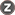Volume 6, Issue 2 (Journal OF Welding Science and Technology 2020)                   2020, 6(2): 65-76 | Back to browse issues page

BibTeX | RIS | EndNote | Medlars | ProCite | Reference Manager | RefWorks
Send citation to:Yousefieh M, Jabbari A. Modeling of temperature in friction stir welding of duplex stainless steel using multivariate lagrangian methods, linear extrapolation and multiple linear regression. Journal title 2020; 6 (2) :65-76
URL: http://jwsti.iut.ac.ir/article-1-329-en.html
Abstract:   (3153 Views)
In this study, the temperature in friction stir welding of duplex stainless steel has been investigated. At first, temperature estimation was modeled and estimated at different distances from the center of the stir zone by the multivariate Lagrangian function. Then, the linear extrapolation method and multiple linear regression method were used to estimate the temperature outside the range and center of the stir zone. Temperature estimation is based on three parameters rotational speed, welding speed and distance from the center of stir zone. In the first method, by generalizing the multivariate Lagrangian method, the multivariate Lagrangian temperature function was generalized according to the above parameters. In the second method, in order to investigate the effect of the variables in the regression model, a comparison of two complete models and a reduced model based on the sum of squares errors was used. Then, by analyzing the multiple regression equations governing the output variable, a multiple linear regression function was introduced. Since the temperature of the stir zone is not measurable by the thermocouple, so in general the best fit curve for estimating the function is when the modeling is based on parameters that minimize the error function.To implement the multiple linear regression method, the error function was introduced to minimize the sum of the error squares and the error derivative was calculated in relation to the parameters of tool rotation speed, welding speed and distance from the center of the stir zone. Therefore, multiple linear regression method was considered as the basic method and as a criterion with other methods. According to the results obtained from the prediction in the center of the stir zone, the temperature difference in all three methods is desirable and negligible. The maximum temperature difference of multiple linear regression method with multivariate Lagrangian method in all nodes was 18.8 oC and multiple linear regression method with linear extrapolation method was 26.36 oC. Therefore, the multivariate Lagrangian interpolation method is less different than the linear extrapolation method in the center of the stir zone and is more accurate.Rights and permissionsThis work is licensed under a Creative Commons Attribution-NonCommercial 4.0 International License.